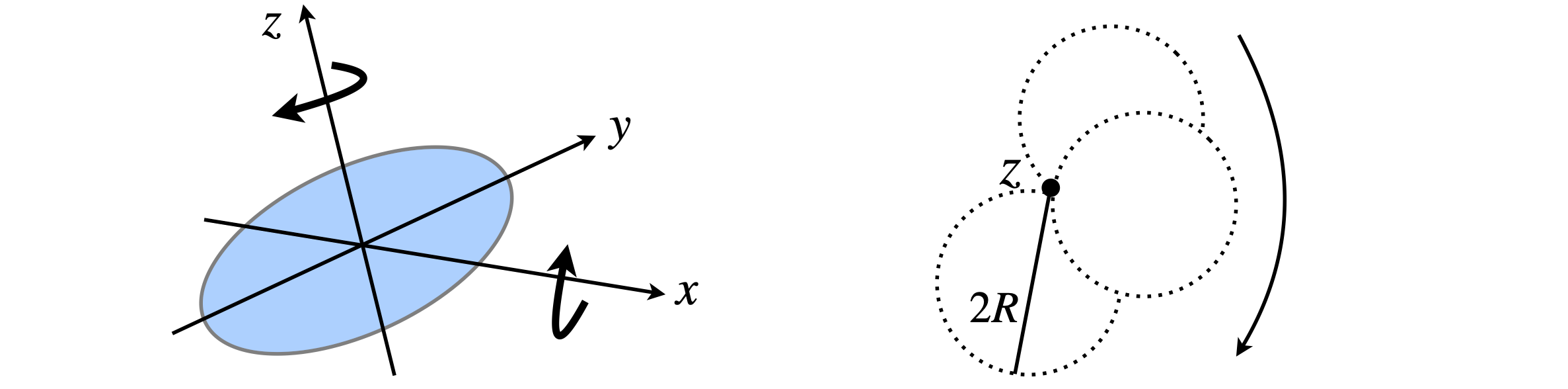# Questions 59 - 62#

## Q59 XeF$$_4$$ moments of inertia.#

Calculate the moments of inertia of the planar molecule XeF$$_4$$

(a) about the C$$_4$$ symmetry axis and

Assume each bond length is $$2 \unicode{x212B}$$ .Figure 67 XeF$$_4$$ and two rotation axes.

## Q60 Loop of wire#

A circular loop of wire is formed by n rigidly connected masses with radius $$R$$ of total mass $$M$$. Explain the different values obtained for the moment of inertia,

(a) About an axis z, through the centre of the loop,

(c) About an edge and parallel to $$z$$.
Strategy: For a planar body to simplify the calculation, use the perpendicular axes theorem $$I_z = I_x + I_y$$ and by symmetry $$I_x = I_y$$.